# Abel's theorem on convergence of power series

Jump to: navigation, search

## Statement

Consider a power series:$\! \sum_{k=0}^\infty a_k(x - \mu)^k$

Suppose that the power series converges to a function$f(x)$ on the interval$(\mu - c, \mu + c)$, i.e.,: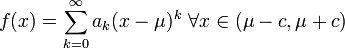$\! f(x) = \sum_{k=0}^\infty a_k(x - \mu)^k \ \forall x \in (\mu - c, \mu + c)$

Then, we have the following:

• If$f$ is defined and left continuous at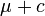$\mu + c$ and the power series converges for$x = \mu + c$, then the value to which the power series converges equals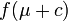$f(\mu + c)$.
• If$f$ is defined and right continuous at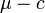$\mu - c$ and the power series converges for$x = \mu - c$, then the value to which the power series converges equals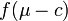$f(\mu - c)$.

Note that Abel's theorem applies even for conditional convergence at endpoints. In fact, that is where it is most helpful in proving things that are not otherwise obvious. Specifically, what it says is that if we can establish conditional convergence at endpoints, and we know the function being converged to on the interior of the interval, we can extend that function to the boundary and find the value at the endpoint of the interval.

In particular, it tells us that for an infinitely differentiable function such that the Taylor series of the function converges to the function on the interior of its interval of convergence, and the Taylor series converges conditionally at one or both of the endpoints of the interval of convergence, then it converges to the function.

Abel's theorem is typically applied in conjunction with the alternating series theorem which is used to show the conditional convergence at one or both endpoints.

## Examples

### Taylor series examples

For simplicity, we discuss the examples below for power series centered at 0, i.e.,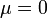$\mu = 0$.

Function Taylor series, also its power series Radius of convergence of power series Endpoints at which the function converges conditionally Consequence of Abel's theorem$x \mapsto \ln(1 + x)$$x - \frac{x^2}{2} + \frac{x^3}{3} - \dots$ 1 1 only (does not converge at -1)
We get this from the alternating series theorem$\ln 2$ is the value of the series at 1. Thus:$\ln 2 = 1 - \frac{1}{2} + \frac{1}{3} - \dots$
arc tangent function$\arctan$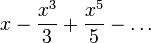$x - \frac{x^3}{3} + \frac{x^5}{5} - \dots$ 1 both -1 and 1
We get this from the alternating series theorem$\pi/4$ is the value of the series at 1. Thus: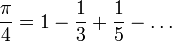$\frac{\pi}{4} = 1 - \frac{1}{3} + \frac{1}{5} - \dots$
We also get a similar statement at -1, but it is equivalent to the above via multiplication of both sides by -1.